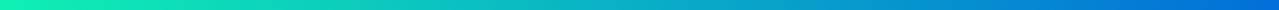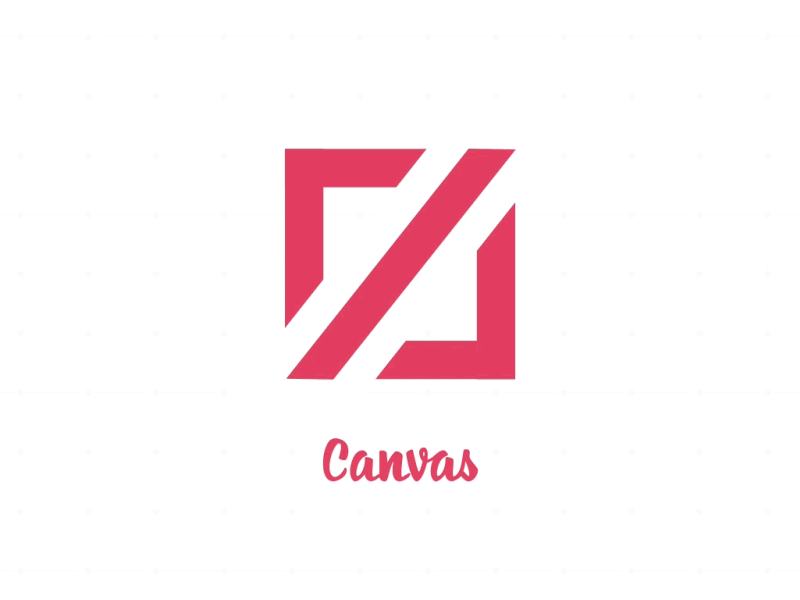Abhi Author of Anicode.in. Passionate full-stack web developer.

# Learn to create awesome 3D Webgl cubix animation!This post is all about programming a gradients based 3d cubix animation using webgl as a renderer and i had explained building it line by line!

so as to make it easy to understand while keeping it simple as 2+2 !

i know you have many questions such as:

• What does use strict do?

• Should I use use strict?

• What is strict mode?

• When would you not use strict mode?

It is not a statement, but a literal expression, ignored by earlier versions of JavaScript. The purpose of “use strict” is to indicate that the code should be executed in “strict mode”.## Where can we place it ?

Strict Mode is a feature that allows you to place in a program, or a function.# Html markup for our code!

There is nothing enough to learn in html & css over here so we will just mark it up and follow our tutorial on javascript’s part!``````1
2
<script src="https://cdnjs.cloudflare.com/ajax/libs/p5.js/0.6.0/p5.min.js"></script>
<script src="https://cdnjs.cloudflare.com/ajax/libs/p5.js/0.8.0/addons/p5.dom.min.js"></script>
``````

Now, lets style our cubixo!# CSS part of our code.

``````1
2
3
4
5
6
7
8
9
body {
margin: 0;
background: rgb(0,0,0);
}

canvas{
border: 1px solid #f00;
}

``````# Let’s start our main part for which you have come for.I guess we have discussed the use of `use strict` earlier!

``````1
"use strict";
``````

# Declaring the constants

Lets declare the variables.

``````1
const { PI: π } = Math;
``````

# Declaring the variables

``````1
2
3
4
let θ = 0;
let w = 24, h;
let offset,ma;
let maxD;
``````

In the code w & h are cube dimensions while offset, ma are angles.

hope all of them are clear to you!## Setting up the functions

Here will create a function named `setup()` which will create a canvas of size `350x350`.

``````1
2
3
4
5
6
function setup() {

createCanvas(350, 350, WEBGL);
ma = atan(cos(π/4));
maxD = dist(0, 0, 200, 200);
}
``````

## Main function

Now, we will make a `draw()` function which will plot our cubes on the canvas.

``````1
2
3
4
5
6
7
8
9
10
11
12
13
14
15
16
17
18
19
20
21
22
23
24
25
26
27
28
29
30
function draw() {

background(0);
orbitControl();
ortho(-400, 400, 400, -400, 0, 1000);
rotateX(ma);
rotateY(-π/4);
offset = 0;

for(let z=0; z<height; z+=w) {
for(let x=0; x<width; x+=w) {
push();

let d = dist(x, z, width/2, height/2);
offset = map(d, 0, maxD, -π, π);

//calculating the angle
let θ1 = θ + offset;

h = map(sin(θ1), -1, 1, 100, 300);
translate(x-width/2, 0, z-height/2);
normalMaterial();
box(w-2, h, w-2);
pop();
}
}

θ = frameCount/10;
}
``````

Let us understand what this function is in a simple manner.

The Orbit control allows the camera to orbit around a target, using the orthographic projection.

Then we will adjust the viewing angle for our canvas in a webgl. Next is Calculating the offset which will be based on distance from center.

Last is increasing the angle a bit for each of the frame by using (θ = frameCount/10)

## Last function!

We should prevent the webgl console from poping up while running the animation.

``````1
2
console.log=()=>{};

``````

This is just a simple arrow function which we used to close the console logs.# Conclusion

Anicode.in! ![Alt Text](https://dev-to-uploads.s3.amazonaws.com/i/860z6owc1uzpylhe6imk.jpg) </center> Hope you learned something amazing! Don't forget to view more such interesting tutorials grab them on [anicode.in](https://anicode.in) ![anicode.in](https://dev-to-uploads.s3.amazonaws.com/i/1cxmrvps5piraagsnbl7.JPG)

comments powered by Disqus